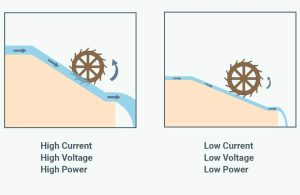# Amps, Volts, Watts, and Watt-hours: Explaining electricity and its measurement units

When you look at your utility bill, your electricity usage is measured in kWh, which stands for kiloWatt-hours (1000 Watt-hours) and is what your utility company charges you for at the end of the month. However, when you look at the electrical specifications of an appliance, a solar…# Energy consumption calculator: Easily calculate the electricity usage of your appliances

Below, you’ll find our Energy Consumption Calculator, which helps you estimate your energy consumption in both Watt-hours (Wh) and kiloWatt-hours (kWh) based on: The appliances you use. Their Power Usage (in Watts). Their daily usage duration (in hours). The number of days in which you use each appliance.…# What size fuse for 300w solar panel?

Fuses and circuit breakers are what you call overcurrent protection devices (or OCPDs for short), and their job is to disconnect the circuit in case the current (in Amps) exceeds a certain threshold. This allows them to protect the wires and the equipment if – for any reason…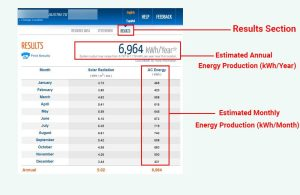# How much does a 5kw solar system produce?

The 5kW (5000 Watts) rating on a solar system means that, provided enough direct sunlight, the system could potentially produce 5000 Watts of power. But the actual amount of power that a system of this size produces is not constant and will fluctuate throughout the day. For example,…# How much electricity does a 4kw solar system produce?

Electricity has many measurement units, such as: Volts, which measure Electrical Potential, or simply voltage. Amps, which measure Electrical Current. Watts or kiloWatts, which measure Electrical Power. Watt-hours or kiloWatt-hours, which measure Electrical Energy. The 4kW (4000W) rating of a solar system means that, provided there’s enough direct…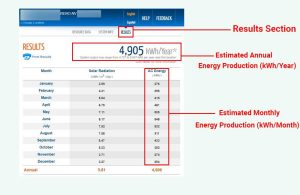# How much power does a 3kw solar system produce?

Unlike Energy, which is accumulative and is measured in Watt-hours (Wh) or kiloWatt-hours (kWh), Power is instantaneous and is measured in Watts (W) or kiloWatts (kW). Power measures the rate at which Energy is being generated. For example, a 3kW (3000 Watt) solar system is capable of producing 3000…# How to calculate battery capacity for solar system?

In a solar system, a battery bank has one important job, and that is to offset 100% of your energy usage. So naturally, when sizing a battery bank for your solar system, your energy usage, among other things, should be taken into consideration. If sized improperly, the battery…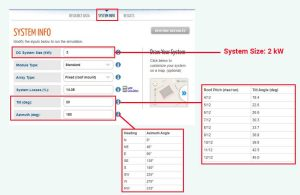# How much power does a 2kw solar system produce?

In the summer, with the right weather conditions, a 2kW (2000 Watts) solar system could produce up to 2 kiloWatts (or 2000 Watts) of power, or even more in some cases. However, what really matters at the end of the day, literally, is not the amount of power…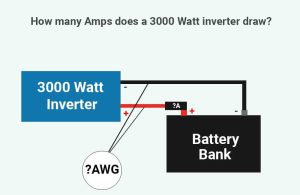# How many amps does a 3000 watt inverter draw?

To find the proper wire and fuse (or circuit breaker) sizes for your 3000 Watt inverter, you’ll need to calculate the maximum amp draw of the inverter. This maximum amp draw will generally depend on 2 factors: The efficiency of your inverter. The lowest battery voltage at which…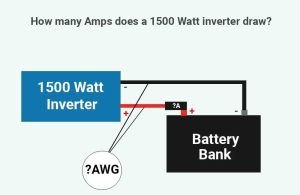# How many amps does a 1500 watt inverter draw?

The amount of current (Amps) that a 1500 Watt inverter draws will mainly depend on the voltage of the battery bank (12V, 24V, or 48V), and the power usage (Watts) of the AC load. However, the wires and over-current protection devices (fuses and circuit breakers) used for the…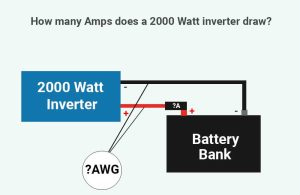# How many amps does a 2000 watt inverter draw?

The maximum amount of Current (Amps) that a 2000 watt inverter is capable of drawing from the battery will mainly depend on 2 factors: The conversion efficiency of the inverter The voltage rating of the battery bank And in order to size the wires and the fuse (or…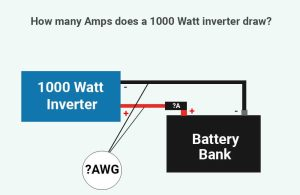# How many amps does a 1000 watt inverter draw?

In this article, I discuss the amount of Current (Amps) that a 1000 Watt inverter is capable of pulling from the battery and explain how to use the voltage of your battery bank and the efficiency of your inverter to determine this amount of current. I also discuss…